# Organizing Data Into Matrices

 Would You Rather Listen to the Lesson?When algebra 2 students familiarize themselves with organizing data into matrices, they first learn how to identify the different types of matrices, count the number of rows and columns in matrices, as well as judge the order of a matrix.

To facilitate these early introductory lessons on matrices, math teachers can employ a variety of teaching strategies. To help out, we’ve created a list of such strategies. Use them in your classroom and see matrix concepts stick for good!## Strategies to Teach Organizing Data Into Matrices

### What Are Matrices?

You can start your lesson on organizing data into matrices by defining what a matrix is. Simply put, a matrix represents a collection of information stored or arranged in an orderly fashion. A matrix represents an array of numbers. Provide an example, such as: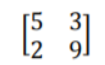Point out that we can have one matrix, or if it’s in plural, several matrices. You can also add that we can perform different operations with matrices, such as multiplication, addition, etc., but this is something that students will learn in more depth in the next classes.

### What Is the Order of a Matrix?

Explain that the order of a matrix represents the number of rows × the number of columns in a given matrix. So if a matrix has y rows and z columns, its order will be y × z (point out that we read this as ‘y by z’). The number of rows and columns are the dimensions of a matrix.

So once children are comfortable with counting the number of rows and columns in a given matrix, they should also be able to determine the order of this matrix. Write a few examples of matrices on the whiteboard and explain their order: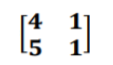In this first example, we can observe that the matrix has two rows and two columns. Thus, we say that its order is 2 × 2.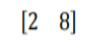In the second example, we can see that there is only one row, but two columns. In other words, its order is 1 × 2.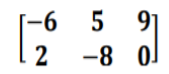Finally, in the third example, the matrix has two rows and three columns. This means that its order is 2 × 3.

### What Is a Matrix Element?

Now that you’ve explained what rows and columns are and how to judge the order of a matrix, you can move on to defining what a matrix element means. A matrix element is simply one of the entries in a matrix.

We can identify every element in a matrix by naming the row and column in which this element is present. We do this by using subscript numbers, where the first subscript represents the row number and the second subscript represents the column number.

Provide an example, such as the matrix G below: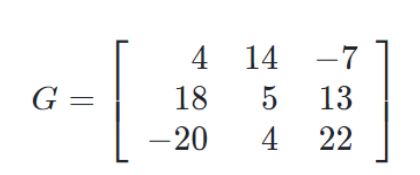Explain to students that in order to find the element g21, we’ll start by looking at the subscript numbers. The first number is 2, so this will be the row number as mentioned earlier; whereas the second number is 1, which indicates the column number.

In other words, the element is the entry in the second row and first column, which corresponds to 18. So g21 = 18.

You can also use highlighters to help students visualize the process of finding the entry. Point out that the easiest way to find the element is by finding the intersection between the two lines shown below.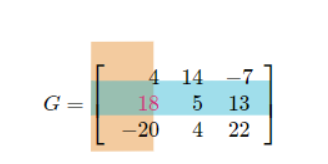If you have the technical means in your classroom, you can also complement your lesson by incorporating multimedia materials, such as videos. For instance, you can use this video as an introduction to matrices to illustrate what matrices are and what the order of a matrix represents.

Moreover, use this video to familiarize students with what matrix elements are. The video contains step-by-step instructions and examples of how to represent the different elements of a given matrix. You can also check out our free resources for adding and subtracting matrices.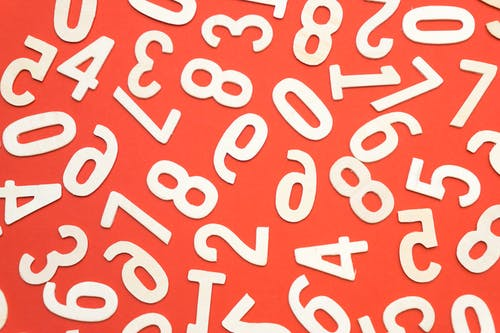## Activities to Practice Organizing Data Into Matrices

### Matrix Dimensions Game

This is a brief online activity that will help students improve their skills at determining the dimensions of a given matrix. To use this activity in your classroom, make sure there is a suitable technical device for each student.

Pair students up and provide instructions for the game. Explain that students are presented with different matrices, and they have to identify the order of each matrix and represent it with the proper notation. If they get stuck, they can watch a video or use a hint.

Each student works individually. Allow a few minutes for students to complete the exercises. When the time is up, students in each pair compare their final scores. The one with the highest score wins the game.

### Matrix Elements Game

This is a short online game that will help students sharpen their ability to identify elements in a given matrix. To use this game in your classroom, make sure there is an adequate technical device for each student.

Students work individually, which makes the game suitable for homeschooling parents as well. Explain that students are presented with different matrices, where they have to correctly identify the required element. If they get stuck, they can also watch a video or use a hint.

Allow a few minutes for students to complete the exercises. When the time is up, allow space for discussion and reflection. Are elements harder to identify in matrices with more rows and columns? Which elements were the hardest to identify? Why?

### Where Am I? Matrix Game

This game is a fun activity to review students’ knowledge of rows and columns in a matrix and matrix element identification. This is a fast-paced game. To implement it in your classroom, you’ll need sheets of paper, matrix element task cards, and markers.

Write one large matrix with several rows and columns on each sheet of paper (make sure that the matrix is different on each sheet). Then, write 10 matrix elements on four different task cards for each matrix on the sheet. You can also laminate the sheets and cards.

Divide students into small groups of 3 or 4. Hand out one matrix sheet to each group and 10 matrix element task cards. Students are supposed to work together with the other members of their group to quickly identify where exactly the required matrix element is on the sheet.

The sheet and task cards remain face down until you give students the signal to start the game. Set a timer for a few minutes and launch the game. When the time is up, the group with the biggest number of correctly identified matrix elements wins the game.

## Before You Leave…

If you like these strategies and activities for teaching organizing data into matrices and you’re looking for more algebra 2 resources, make sure to sign up for our emails to receive loads of free lessons and content!

In addition, you can sign up for a membership on MathTeacherCoach or head over to our blog, where you’ll find more math materials for kids of all ages. You’ll discover that with the resources we offer, teaching math is super fun and easy!